Syllogism Questions for RRB NTPC Set-3 PDF

0
562

Syllogism Questions for RRB NTPC Set-3 PDF

Download RRB NTPC Syllogism Questions and Answers 2019 Set-3 PDF. Top 15 RRB NTPC Syllogism questions based on asked questions in previous exam papers very important for the Railway NTPC exam.

Question 1: There are two/ three statements are given below, followed by four conclusions I, II, III & IV which may be drawn from the statements. You have to consider the statements to be true even if they seem to be at variance from commonly known facts. You have to decide which one of the given conclusions is/are drawn from the given statements.
Statements
I: All Televisions sold in that shop have high standard
II: Some Onida Televisions are sold in that shop
Conclusions
I: All Televisions of high standard are manufactured by Onida
II: Some of the Onida Televisions are of high standard
III: None of the Onida Televisions is of high standard
IV: Some of the Onida Televisions of high standard are sold in that shop

a) All I, II, III and IV follow

b) Only I follows

c) Only II and IV follow

d) Only III follows

Question 2: Three statements are given followed by three conclusions. You have to consider the statements to be true even if they seem to be at variance from commonly known facts. You have to decide which one of the given conclusions is definitely drawn from the given statements.
Statements
1. All mangoes are fruits
2. All fruits are vegetables
3. Some vegetables are grass
Conclusions
1. Some fruits may be grass
2. No mango is grass
3. All grass is vegetable

a) Only 1

b) Only 2

c) Only 1 and 2

d) All of these

Question 3: Three statements are given followed by three conclusions. You have to consider the statements to be true even if they seem to be at variance from commonly known facts. You have to decide which one of the given conclusions is definitely drawn from the given statements.
Statements:
1) All biscuits are chocolates
2) All chocolates are snacks
3) No snack is a meal
Conclusions:
1) Some snacks are biscuits
2) Some meals are chocolates
3) All meals are biscuits

a) Only 1

b) Only 2

c) 1 and 2

d) 1,2 and 3

Question 4: There are two/ three statements are given below, followed by four conclusions I, II, III & IV which may be drawn from the statements. You have to consider the statements to be true even if they seem to be at variance from commonly known facts. You have to decide which one of the given conclusions is/are drawn from the given statements.
Statements
I: All Puppies are Dogs
II: All Dogs are Cats
III: No Cat is Mouse
Conclusions
I: Some Dogs are Puppies
II: Some Cats are Dogs
II: No Mouse is a Cat
II: All Puppies are Cats

a) All I, II, III and IV follow

b) Only I follows

c) Only I and II follow

d) Only III follows

Question 5: Three statements are given followed by three conclusions. You have to consider the statements to be true even if they seem to be at variance from commonly known facts. You have to decide which one of the given conclusions is definitely drawn from the given statements.
Statements:
1) All mangoes are apples
2) All apples are fruits
3) No fruit is vegetable
Conclusions:
1) Some fruits are mangoes
2) Some vegetables are apples
3) All mangoes are fruits

a) Only 1

b) Only 3

c) 1 and 3

d) 1, 2 and 3

Question 6: Some statements are given which are followed by two or more conclusions/assumptions- I, II, III. You have to consider the statements to be true, even if it seems to be at variance from the commonly known facts. You have to decide which of the given conclusions/assumptions can definitely be drawn from the given set of statements.
Statements:
I) All males are dogs
II) Some dogs are pugs.
III) No male is pug.
IV) Some males are professors.
Conclusions:
I) All professors are Males
II) No pug is professor
III) Some pugs are not dogs

a) Only III follows

b) Both I and III follow

c) Both I and II follow

d) All conclusion follow

Question 7: Instructions: Two or three statements are given followed by two are three conclusions. You have to consider the statements to be true even if they seem to be at little variance with the commonly known facts. You have to choose which of the given conclusions definitely follow.
Statements:
1) Some raccoons are lizards
2) All raccoons are monkeys
3) Some monkeys are donkeys
Conclusions:
1) Some donkeys are lizards
2) Some lizards are monkeys
3) Some donkeys are raccoons

a) Conclusion 1 follows

b) Conclusion 2 follows

c) Conclusion 1 and 2 follow

d) Conclusio 2 and 3 follow

Instructions

In each of the following questions two/ three statements are given followed by two conclusions numbered I and II. You have to take the given statements to be true even if they seem to be at variance from commonly known facts. Read both the conclusions and then decide which of the given conclusions logically and definitely follows from the given statements disregarding commonly known facts.

Question 8: Statements
All books are journals.
All diaries are journals.
Conclusions:
I. All Journals are books.
II. Some diaries being books is a possibility

a) Either conclusion I or Conclusion II follows

b) Only conclusion I follows

c) Both Conclusion I and Conclusion II follow

d) Neither Conclusion I nor Conclusion II follows

e) Only Conclusion II follows

Question 9: Statements
No fruit is vegetable
All potatoes are vegetables
Some fruits are apples.
Conclusions:
I. Some apples are potatoes
II. Some potatoes being fruits is a possibility.

a) Both Conclusion I and Conclusion II follow

b) Only conclusion II follows

c) Either conclusion I or Conclusion II follows

d) Only conclusion I follows

e) Neither conclusion I nor Conclusion II follows

Question 10: Statements
All beans are pulses
All pulses are crops.
No crop is seed
Conclusions:
I. All crops are pulses
II. All beans are crops

a) Only Conclusion II follows

b) Neither conclusion I nor conclusion II follows

c) Either Conclusion I nor Conclusion II follows

d) Only Conclusion I follows

e) Both Conclusion I and Conclusion II follow

Instructions

In each of the questions given below, there are three statements given followed by three conclusions numbered I, II and III. You have to take the given statements to be true even if they seem to be at variance from commonly known facts. Read all the conclusions and then decide which of the given conclusions logically follows from the given statements disregarding commonly known facts.

Question 11: Statements:
All buildings are rivers.
All rivers are jungles.
All jungles are mountains.Conclusions:
I. Some mountains are rivers.
II. Some jungles are buildings.
III. Some mountains are buildings.

a) Only I and II follow

b) Only I and III follow

c) Only II and III follow

d) All I, II and III follow

e) None of these

Question 12: Statements:Some bulbs are tubes.
Some wires are lamps.
Some wires are tubes.
Conclusions:
I. Some lamps are tubes.
II. Some wires are bulbs.
III. Some lamps are bulbs.

a) None follows

b) Only I follows

c) Only II follows

d) Only III follows

e) Only II and III follow

Question 13: Statements:
Some marbles are sticks.
No stick is garden.Conclusions:
I. Some gardens are marbles.

a) None follows

b) Only I follows

c) Only II follows

d) Only III follows

e) Only II and III follow

Question 14: Statements:
All pictures are walls.
Some walls are rooms.
Some rooms are windows.Conclusions:
I. Some windows are walls.
II. Some windows are pictures.
III. Some rooms are walls.

a) None follows

b) Only I follows

c) Only II follows

d) Only III follows

e) Only II and III follow

Question 15: Statements:
All books are tents.
Some tents are lakes.
All lakes are ponds.Conclusions:
I. Some ponds are books.
II. Some ponds are tents.
III. Some lakes are books.

a) None follows

b) Only I follows

c) Only II follows

d) Only III follows

e) Only II and III follow

As we can check from the diagram.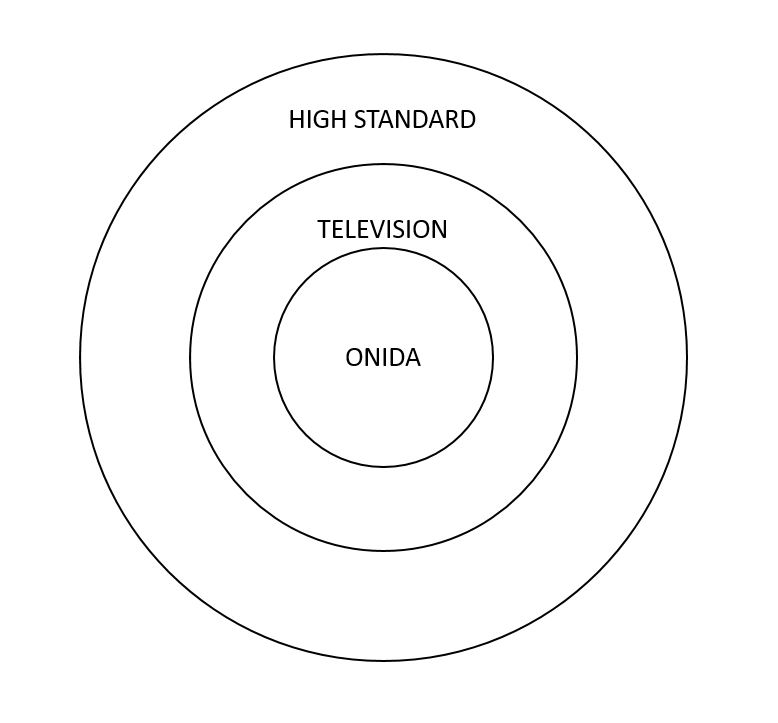Conclusions
I: All Televisions of high standard are manufactured by Onida $\times$
II: Some of the Onida Televisions are of high standard $\checkmark$
III: None of the Onida Televisions is of high standard $\times$
IV: Some of the Onida Televisions of high standard are sold in that shop $\checkmark$
Therefore, all the statements follow. Hence, Option C is correct.

All mangoes are fruits and all fruits are vegetables. Hence all mangoes must be vegetables. Some vegetables are grass.
Now conclusion I says that some mangoes may be grass. The thing to note here is that the conclusion uses the word ‘may’. Hence the conclusion need not be true in all cases. Even if it is true in some cases, that would suffice. Conclusion II says that no mango is grass. This needs to be true in all cases. If we can have one cases where this is not being followed than the conclusion does not hold true. Conclusion III says that all grass is vegetable. This should always be true.
Among the given conclusions only conclusion I is true. This can be validated by the following venn diagram.Biscuits, chocolates, snacks and meals can be represented as shown in the diagram.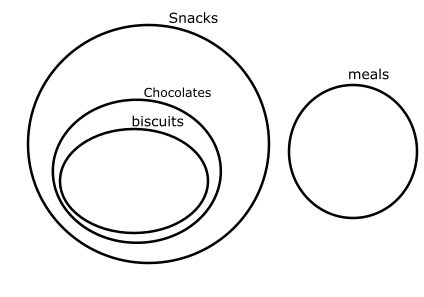From the figure we can say that some snacks are biscuits. Hence, conclusion 1 is true
No meal is a snack. Hence, conclusion 2 is false.
No meal is a biscuit. Hence, conclusion 3 is false.

As we can check from the diagram.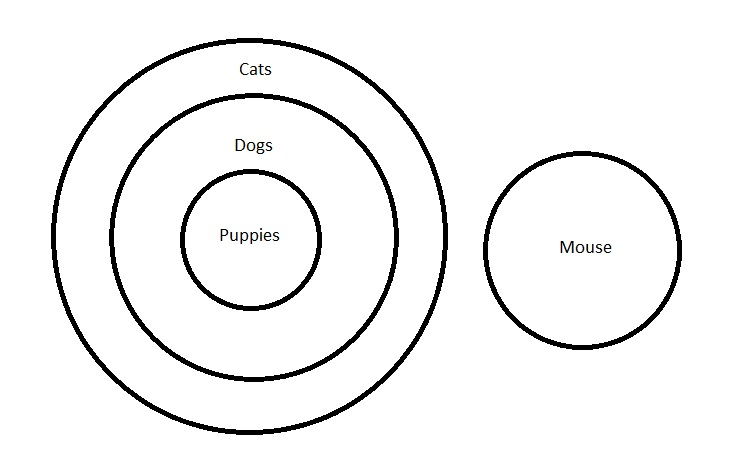Conclusions
I: Some Dogs are Puppies $\checkmark$
II: Some Cats are Dogs $\checkmark$
II: No Mouse is a Cat $\checkmark$
II: All Puppies are Cats $\checkmark$
Therefore, all the statements follow. Hence, Option A is correct.

Mangoes, apples, fruits and vegetables can be represented as shown in the figure below.From the figure we can see that, some fruits are mangoes. Thus conclusion 1 is true.
We can see that no vegetable is an apple. Thus conclusion 2 is not true.
Similarly we can see that all mangoes are fruits. Thus conclusion 3 is true.
Hence Option C.

Consider the following figure-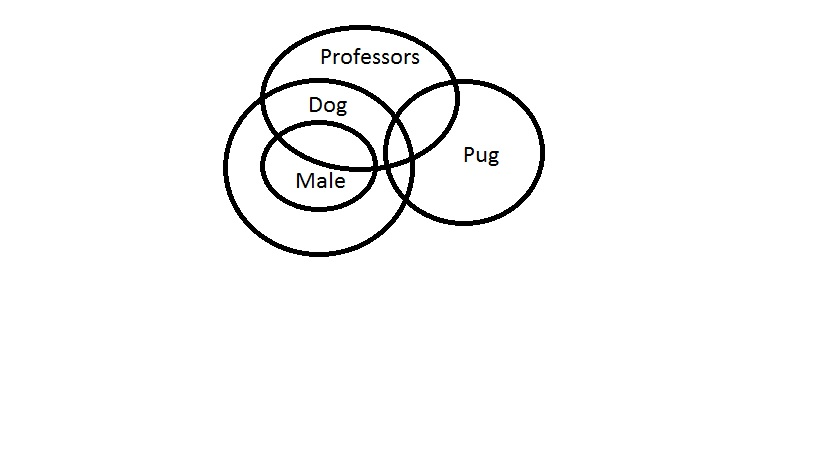We can see that all professors need not be males. So, I is incorrect.
II is also incorrect as we can see that an overlap between professor and pugs is possible.
III is always true since some pugs are not dogs.
Thus, A is the correct answer.

The following venn diagram can be drawn based on the given instructions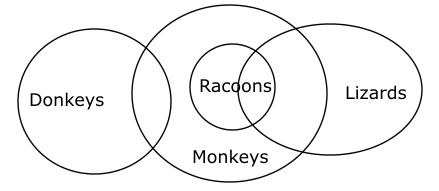Given that some monkeys are donkeys this doesn’t necessarily mean that some donkeys are lizards and raccoons.
So, conclusions 1 and 3 are incorrect.

All raccoons are monkeys and some raccoons are lizards ==> some lizards are monkeys.

Therefore, conclusion 2 is correct.

(i) All beans are pulses Universal affirmative (A-type).
(ii) Some fruits are apples particular Affirmative (I-type).
(iii) No crops is seed Universal Negative (E-type).
(iv) Some crops are not seeds Particular Negative (O-type).

All diaries are journals. Some journals are books.
A + I => No conclusionFrom the above diagram it is clear that “some diaries being books is a possibility.”

(i) All beans are pulses Universal affirmative (A-type).
(ii) Some fruits are apples particular Affirmative (I-type).
(iii) No crops is seed Universal Negative (E-type).
(iv) Some crops are not seeds Particular Negative (O-type).

Some apples are fruits. No fruits is vegetable.
I + E => O-type of conclusion
“Some apples are not vegetables.”

All potatoes are vegetables. No vegetable is fruit.
A + E => E-type of conclusion
“No potato is fruit”.

Neither conclusion I nor II follows.

(i) All beans are pulses Universal affirmative (A-type).
(ii) Some fruits are apples particular Affirmative (I-type).
(iii) No crops is seed Universal Negative (E-type).
(iv) Some crops are not seeds Particular Negative (O-type).

All beans are pulses. All pulses are crops.
A + A => A-type of conclusion
“All beans are crops.”
This is conclusion II

All beans are crops. No crops is a seed
A + E => E-type of conclusion
“No bean is a seed.”

All pulses are crops. No crops is a seed.
A + E E-type of conclusion
“No pulse is a seed.”

Consider the following figure-We can see that all the conclusions follow. Thus, D is the answer.

Consider the following figure-We can see that none of the conclusions follow. Thus, A is the answer.

Consider the following figures-From the first figure, we can conclude that I need not be true all the times. Similarly from the second figure, we can conclude that III need not be true all the times. But II is true every time. Thus, the answer is C.

Consider the following figure-We can see that only conclusion III follows. Thus, D is the correct answer.